## Dynamic behaviour of system

12
0
2
publish time: 2020-10-12Charlotte

This example shows how UML diagrams can be used to describe the dynamic behaviour of a system, the structural organization, and interaction among objects. All of this, while considering the time and order in which events happen, thus keeping an eye on the sequence of events and message flows.

See More Related TemplatesThis is an example of a UML diagram about adding events to the calendar.
UML Diagram - Add event to calendar
23
1
0easy diagrams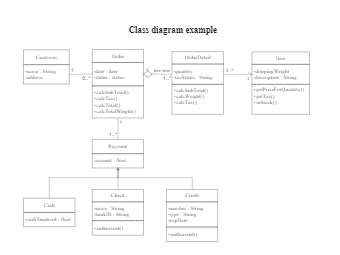This is an example of a uml class diagram.
Class diagram example
203
6
4easy diagrams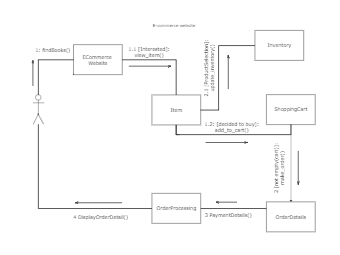This is an example of a UML collaboration diagram.
ecommerce website - UML diagram
24
2
0easy diagrams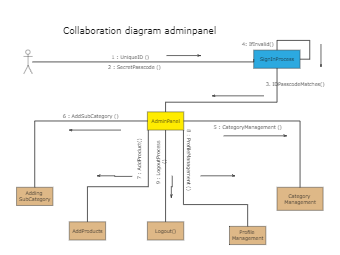11
1
0easy diagrams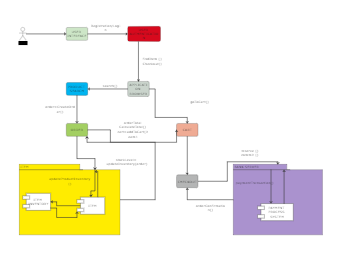This is an example of a UML communication diagram.
Communication diagram example
11
1
1easy diagrams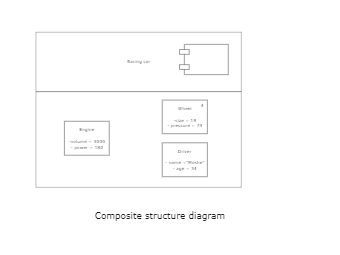This is an example of a composite structure diagram.
Composite structure diagram
3
0
0easy diagrams